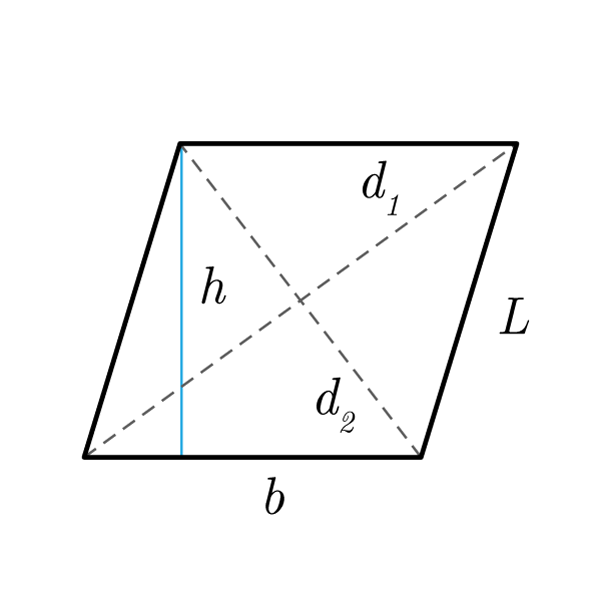# Parallelogram

 Base $$b$$ Height $$h$$ Oblique Side $$S$$ Longer Diagonal $$d_{1}$$ Shorter Diagonal $$d_{2}$$
$$2p = 2b + 2S$$
Perimeter
$$b = \frac{2p - 2S}{2}$$
Base
$$S = \frac{2p - 2b}{2}$$
Oblique Side
$$A = b \times h$$
Area
$$b = \frac{A}{h}$$
Base
$$h = \frac{A}{b}$$
Height

## Definition

A parallelogram is a quadrilater with opposite sides parallel (and congruent).

## Properties

1. Opposite sides are congruent and parallel
2. Opposite angles are congruent, consecutive angles are supplementary (their sum is 180°)
3. Diagonals intersect in their midpoint## Parallelogram Formulas

Data Formula
Perimeter 2p = 2 × b + 2 × S
Area A = b × h
Base b = A / h
Height h = A / b
Base b = (2p - 2 × S) / 2
Oblique Side S = (2p - 2 × b) / 2